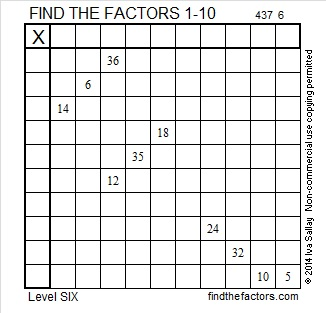# 237 and Level 6

• 237 is a composite number.
• Prime factorization: 237 = 3 x 79
• The exponents in the prime factorization are 1 and 1. Adding one to each and multiplying we get (1 + 1)(1 + 1) = 2 x 2 = 4. Therefore 237 has 4 factors.
• Factors of 237: 1, 3, 79, 237
• Factor pairs: 237 = 1 x 237 or 3 x 79
• Since 237 has no square number factors, its square root cannot be simplified. √237 ≈ 15.395Print the puzzles or type the factors on this excel file: 10 Factors 2014-09-15